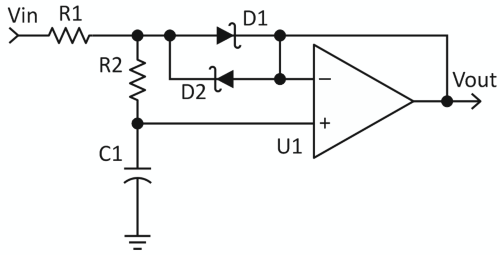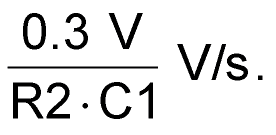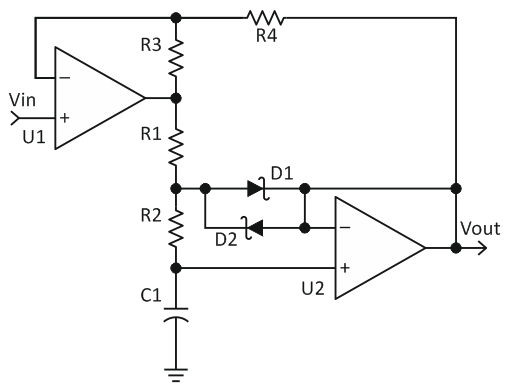# Linear ramp generator uses one op-amp

Einar Abell

EDN

This Design Idea demonstrates a simple way to turn a step input into a ramp of nearly constant slew rate. The basic circuit uses a single op-amp; a circuit built on an integrator will typically require three.

Figure 1 shows the basic circuit.Figure 1. Ramp generator.

The op-amp is wired as a voltage follower, with D1 & D2 clamping the input to one diode drop above or below the output. When the input step-changes by a volt or more, the diodes will keep the voltage across R2 at a nearly constant 0.3 V (because the output will follow any change on C), creating a constant slew rate at the output ofThe output will slew to within 0.3 V of the input and then follow an RC ((R1+R2)C1) curve until reaching a final value equal to VIN. For best results, R2 should be larger than R1, preferably by factor of ten or more. This simple circuit gives best results with fairly large steps, where the slow settling time won't be objectionable.

Figure 2 adds an extra amplifier to make the output slew to within a few millivolts of the input.Figure 2. Improved ramp generator.

Amplifier U1 amplifies the difference between the input and the output so as to keep the diode clamp operating until the output voltage nearly reaches the input. The output will slew to within the diode forward voltage divided by the gain of U1 (that is, 1+(R3/R4)).

If the gain is made too large, the circuit will become unstable. The slew rate of U1 should be at least ten times (the higher the better) the output slew rate times the gain of U1. U1 needs extra headroom to keep D1 & D2 conducting, which usually means restricting the input so that it is more than one volt away from the max/min output swing of U1.

EDN